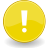# LlChildPrims2List

 LSL PortalLSL Feature Request The described function does not exist. This article is a feature request.
Function: list llChildPrims2List( integer start, integer end );
 REQUEST Function ID 0.0 Forced Delay 10.0 Energy

Returns a list of child prim keys.

 • integer start – start index • integer end – end index

start & end support negative indexes.

## Caveats

• If either start or end are out of bounds the script continues to execute without an error message.
• start & end will form an exclusion range when start is past end (Approximately: start > end).
All Issues ~ Search JIRA for related Bugs

## Examples

```list llChildPrims2List(integer start, integer end){//this probably doesn't work
integer prims = (llGetNumberOfPrims() * !!llGetAttached()) + llGetObjectPrimCount(llGetKey());
integer first = (prims != 1);
integer last = prims - !first;
if(start < 0)
start += first + prims;
if(end < 0)
end += first + prims;

list out;
if(start > end) {
if(end >= last) {
do {
} while(last >= ++first);
} else {
while(first <= end){
}
while(start <= last){
}
}
} else if(end >= first && start <= last) {
if(start < first)
start = first;
if(end > last)
end = last;
do {
} while(end >= ++start);
}
return out;
}
```

```//function list llChildPrims2List( integer start, integer end );